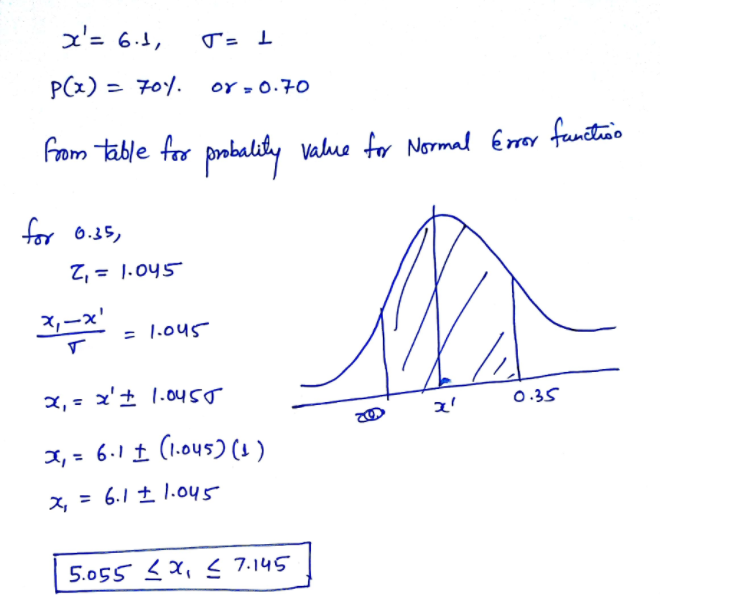# Question Solved1 AnswerMeasurements: A large population of variable x is characterized by its known mean value of 6.1 units and a standard deviation of 1.0 units and a normal distribution. Determine the central range of values containing 70% of the population of x. (Explain how we got P(z1) = 0.35 or z1 = 1.04) x'= 6.1, T= 1 P(x) = 701 OY - 0.70 from table for probalily value for Normal Evor fantino for 0.35 Z, = 1.045 4,-x' = 1.045 T 2,= '1.0455 0.35 z' CO 2. = 6.1 † (1.045) (1) x = 6.1 ± 1.045 5.055 5X, 57.1459FSFBV The Asker · Probability and Statistics

Measurements:

A large population of variable x is characterized by its known mean value of 6.1 units and a standard deviation of 1.0 units and a normal distribution. Determine the central range of values containing 70% of the population of x. (Explain how we got P(z1) = 0.35 or z1 = 1.04)Transcribed Image Text: x'= 6.1, T= 1 P(x) = 701 OY - 0.70 from table for probalily value for Normal Evor fantino for 0.35 Z, = 1.045 4,-x' = 1.045 T 2,= '1.0455 0.35 z' CO 2. = 6.1 † (1.045) (1) x = 6.1 ± 1.045 5.055 5X, 57.145
More
Transcribed Image Text: x'= 6.1, T= 1 P(x) = 701 OY - 0.70 from table for probalily value for Normal Evor fantino for 0.35 Z, = 1.045 4,-x' = 1.045 T 2,= '1.0455 0.35 z' CO 2. = 6.1 † (1.045) (1) x = 6.1 ± 1.045 5.055 5X, 57.145